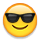# Formula CalculationI am writing calculation formula which I am struggle a little, hoping anyone can help me out.

When writing calculation formula in Excel, we can use ( ) to group the calculation together, but Anaplan does not work the same way or am I work? Is there a way to group calculation together within Anaplan?

Tagged:

•ex. (A +> (D + E) would be shown as A + B > C + D
whereas (A +/ (C + D) would keep the parenthesis.